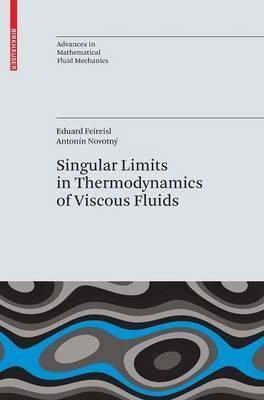# Singular Limits in Thermodynamics of Viscous Fluids

In stock
SKU
BK0051278
ISBN13 9783764388423
Binding PAPERBACK
Author FEIREISL
Language ENGLISH
Publisher SPRINGER VERLAG
Country of Origin India

# 9249.00

Many interesting problems in mathematical fluid dynamics involve the behavior of solutions of nonlinear systems of partial differential equations as certain parameters vanish or become infinite. Frequently the limiting solution, provided the limit exists, satisfies a qualitatively different system of differential equations. This book is designed as an introduction to the problems involving singular limits based on the concept of weak or variational solutions. The primitive system consists of a complete system of partial differential equations describing the time evolution of the three basic state variables: the density, the velocity, and the absolute temperature associated to a fluid, which is supposed to be compressible, viscous, and heat conducting. It can be represented by the Navier-Stokes-Fourier-system that combines Newton's rheological law for the viscous stress and Fourier's law of heat conduction for the internal energy flux.As a summary, this book studies singular limits of weak solutions to the system governing the flow of thermally conducting compressible viscous fluids.